Examples

Chapter 4 Class 9 Linear Equations in Two Variables
Serial order wise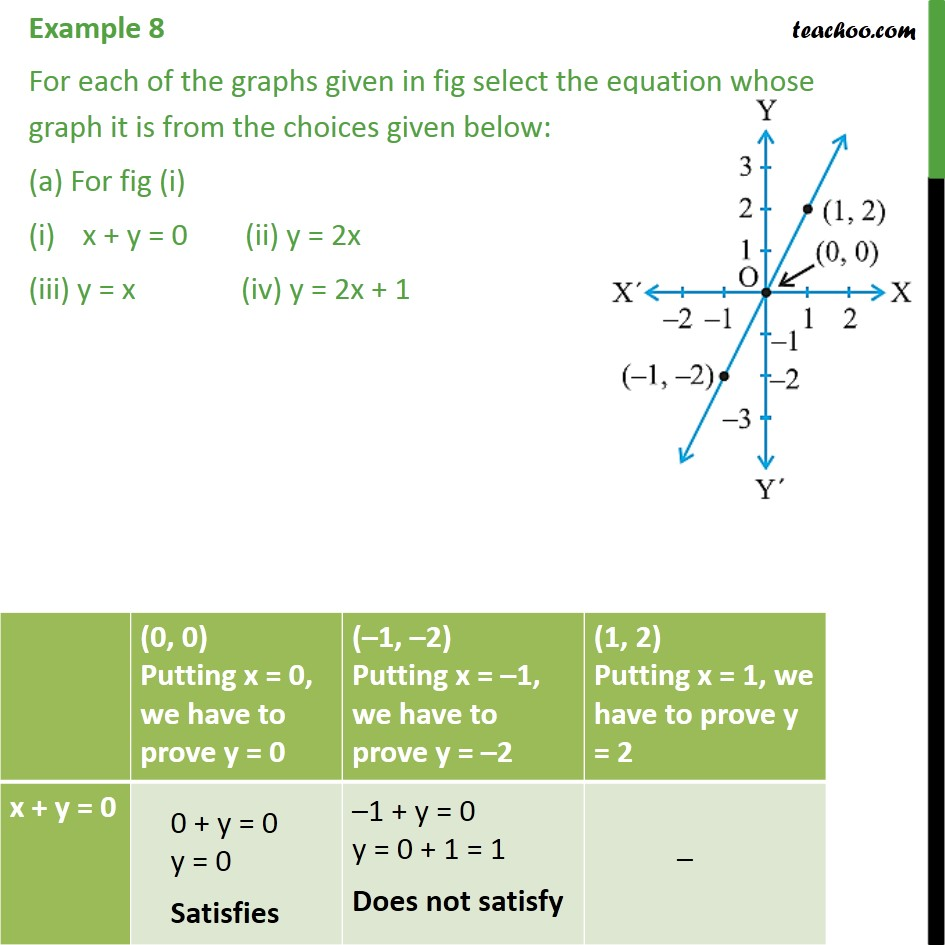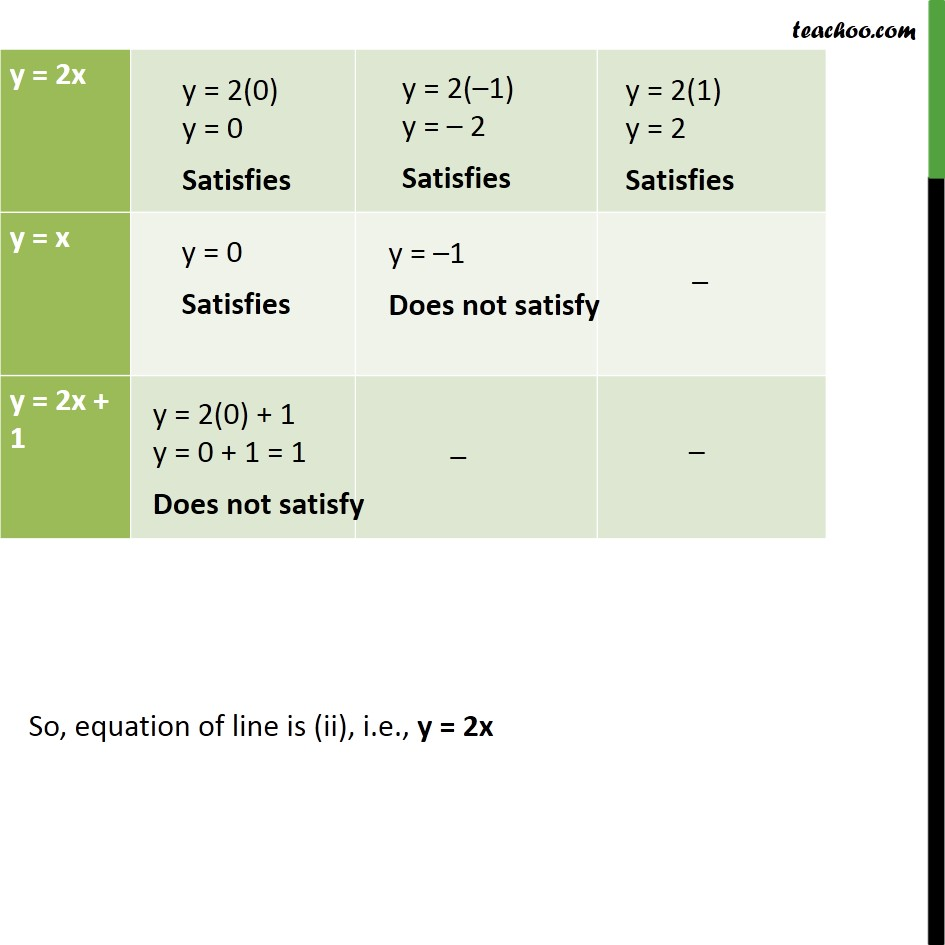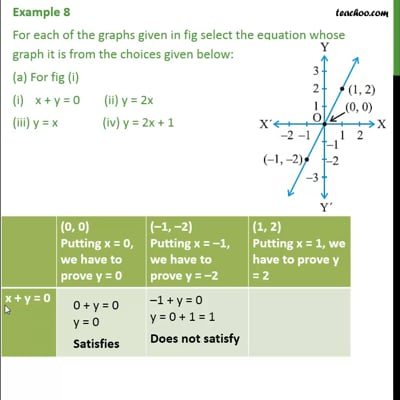This video is only available for Teachoo black users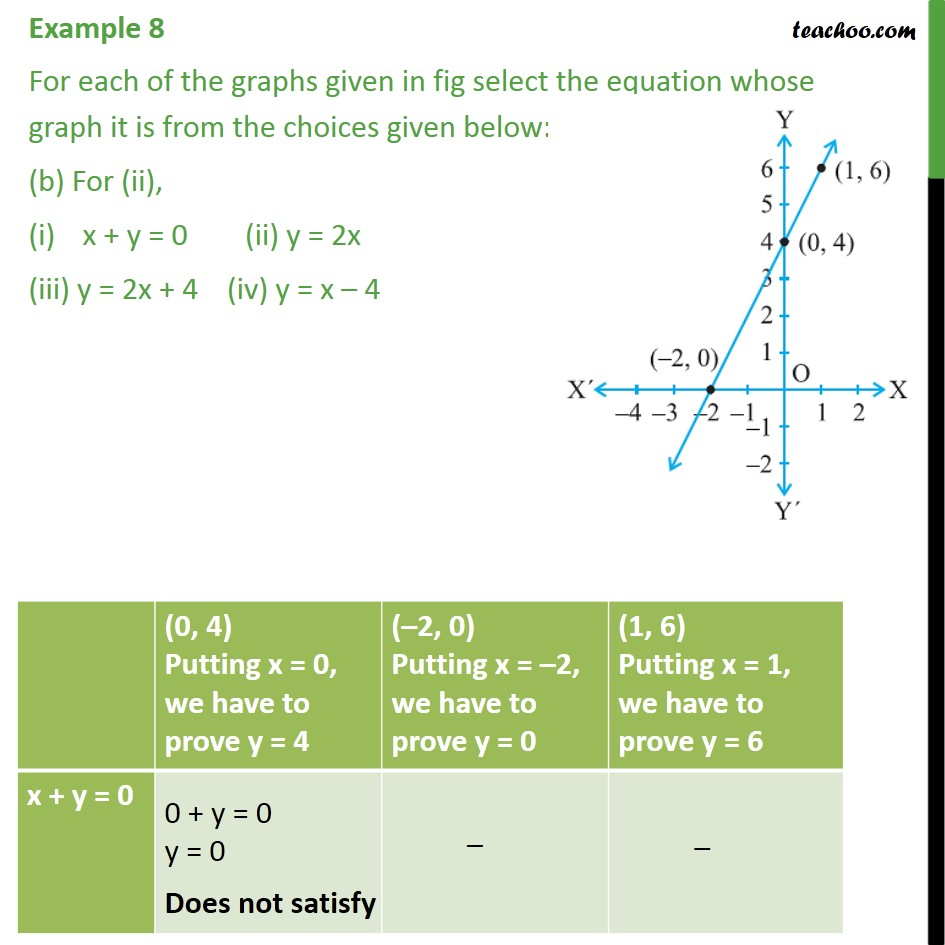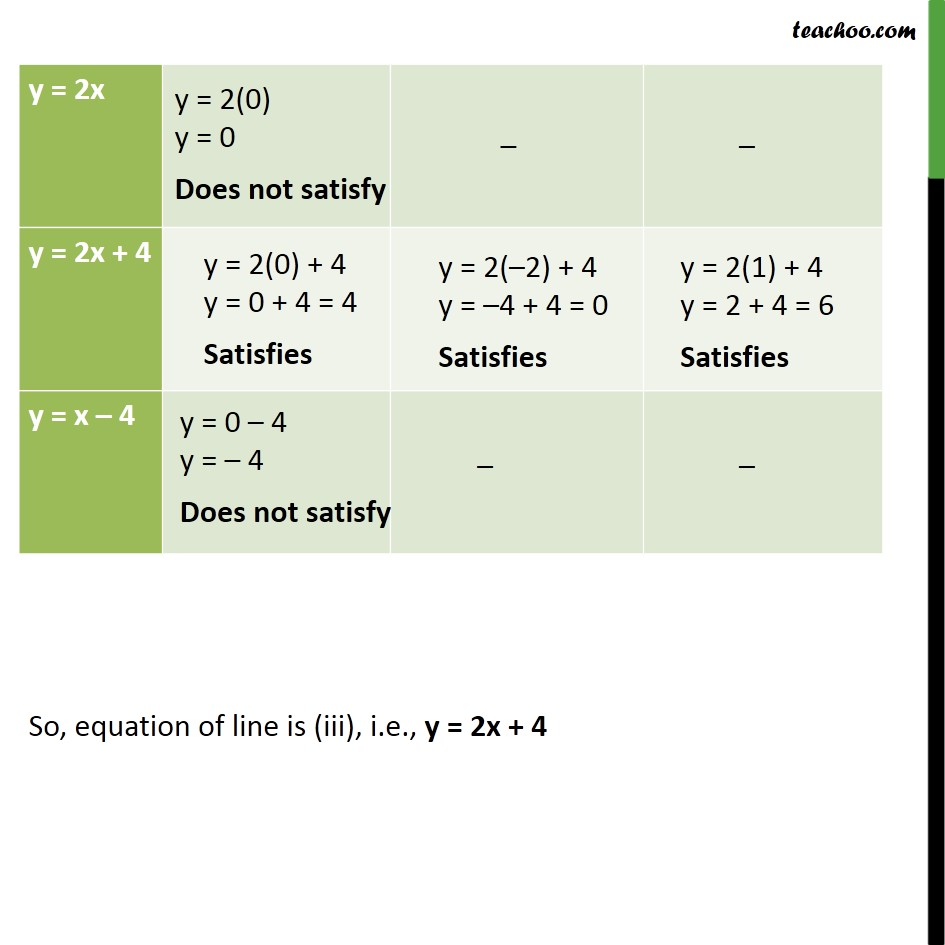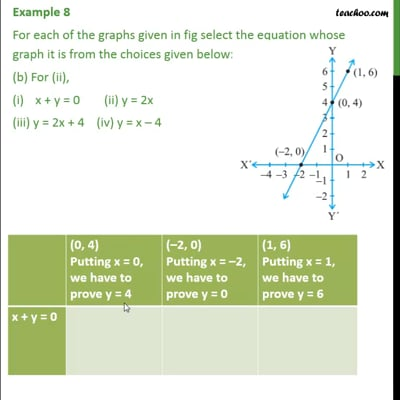This video is only available for Teachoo black users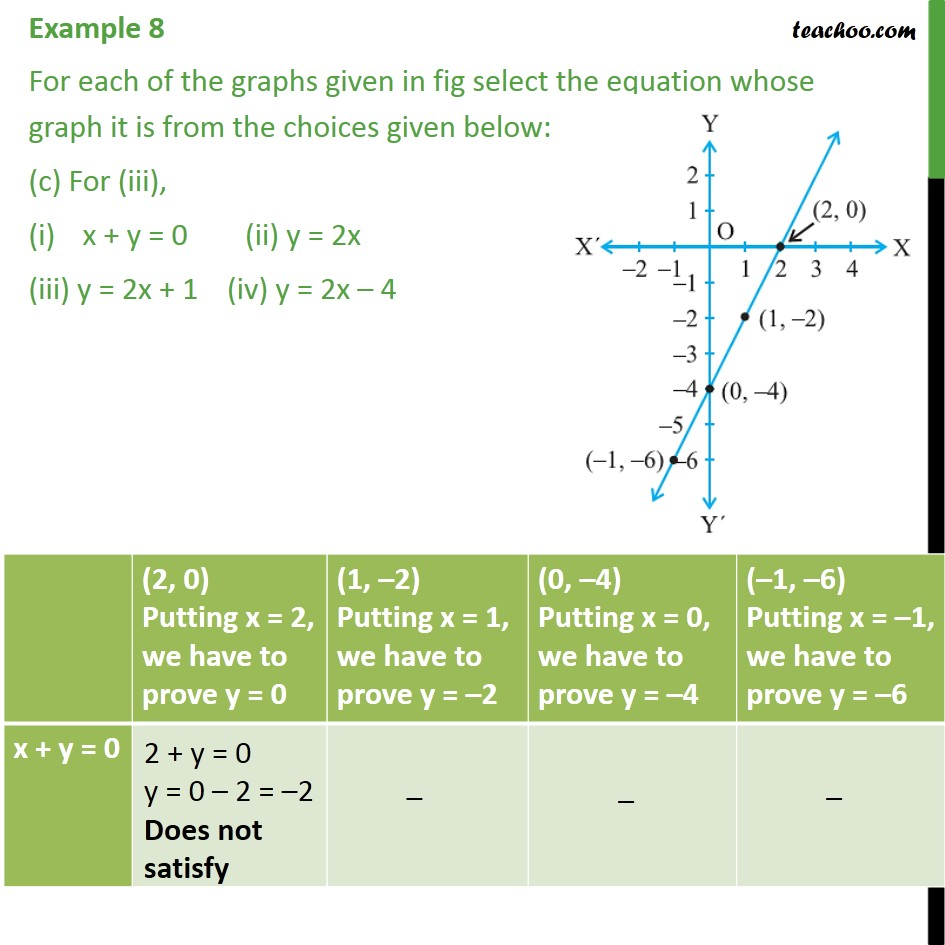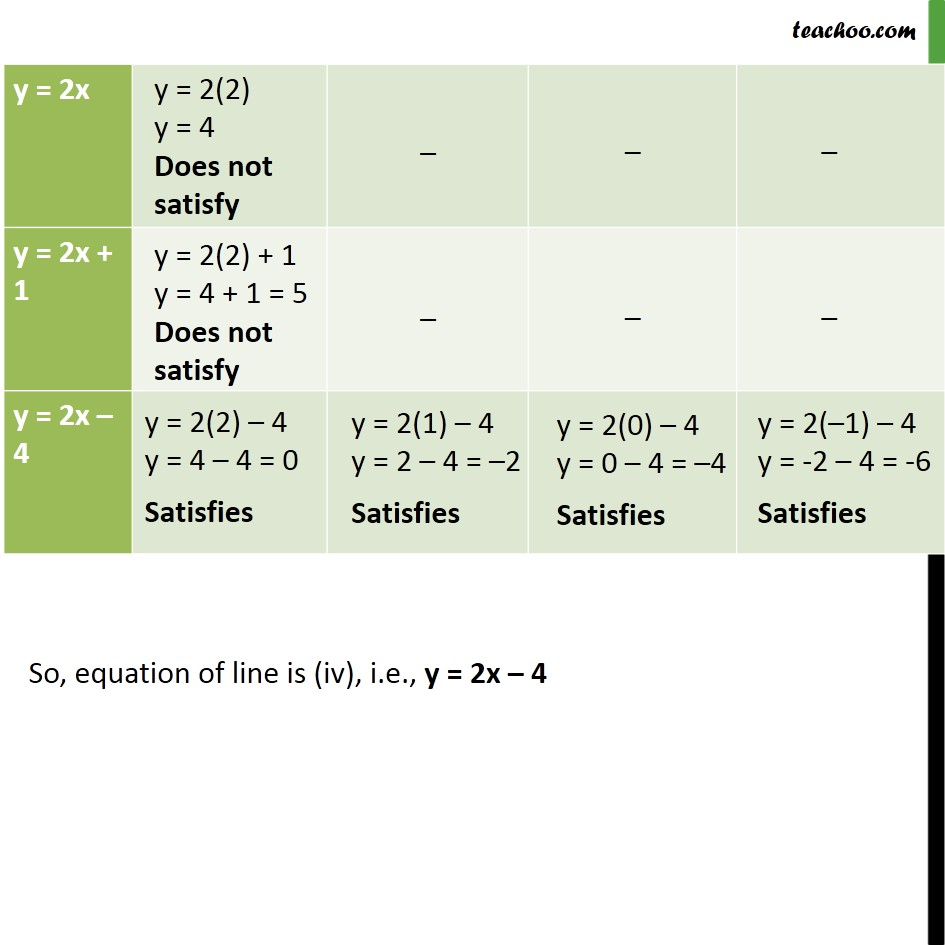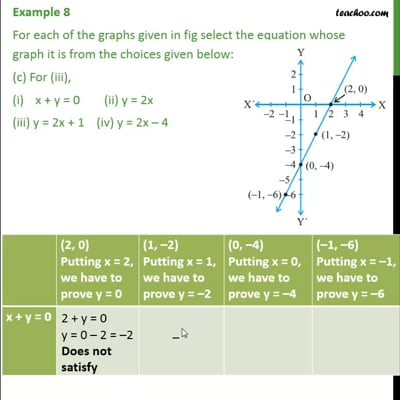This video is only available for Teachoo black users

Solve all your doubts with Teachoo Black (new monthly pack available now!)

### Transcript

Example 8 For each of the graphs given in fig select the equation whose graph it is from the choices given below: (a) For fig (i) x + y = 0 (ii) y = 2x (iii) y = x (iv) y = 2x + 1 So, equation of line is (ii), i.e., y = 2x Example 8 For each of the graphs given in fig select the equation whose graph it is from the choices given below: (b) For (ii), x + y = 0 (ii) y = 2x (iii) y = 2x + 4 (iv) y = x 4 So, equation of line is (iii), i.e., y = 2x + 4 Example 8 For each of the graphs given in fig select the equation whose graph it is from the choices given below: (c) For (iii), x + y = 0 (ii) y = 2x (iii) y = 2x + 1 (iv) y = 2x 4 So, equation of line is (iv), i.e., y = 2x 4